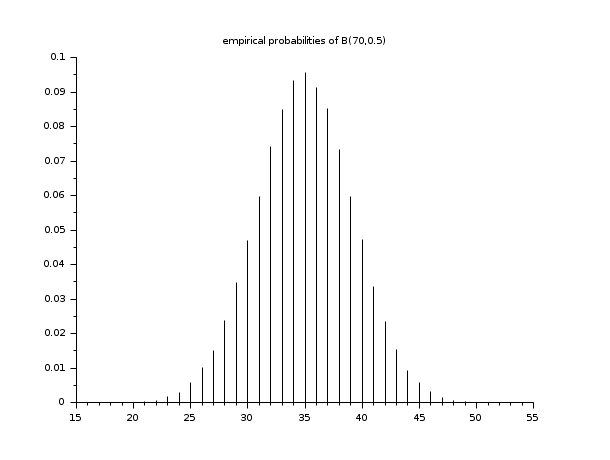Scilab Home page | Wiki | Bug tracker | Forge | Mailing list archives | ATOMS | File exchange
Scilab 5.5.1
Change language to: English - Português - 日本語 - Русский

See the recommended documentation of this function

Aide de Scilab >> Statistiques > Summaries > tabul

# tabul

frequency of values of a matrix or vector

### Calling Sequence

`[m]=tabul(X [,order])`

### Arguments

X

vector or matrix (of real or complex numbers or strings)

order

(optional) a character equal to "d" or "i" (default value "d")

m

a 2 columns matrix (if `X` is a numerical vector or matrix) or a list with 2 members (if `X` is a string vector or matrix).

### Description

This function computes the frequency of values of the components of a vector or matrix `X` of numbers or string characters :

if X is a numerical vector or matrix

then `m` is a two column matrix who contains in the first column the distinct values of `X` and in the other column the number of occurrences of those values (m(i,2) is the number of occurrences of m(i,1)).

if X is a string vector or matrix

then `m` is a list whose first member is a string (column) vector composed with the distinct values of `X` and the second member is a (column) vector whose components are the number of occurrences of those values ( m(i)(2) is the number of occurrences of the string m(i)(1) ).

The optional parameter `order` must be "d" or "i" (by default order="d") and gives the order (decreasing or increasing) the distinct values of X will be sorted.

### Examples

```X = [2 8 0 3 7 6 8 7 9 1 6 7 7 2 5 2 2 2 9 7]
m1 = tabul(X)
m2 = tabul(X, "i")```
```X = ["ba" "baba" "a" "A" "AA" "a" "aa" "aa" "aa" "A" "ba"]
m = tabul(X,"i")```
```n = 50000;
X = grand(n,1,"bin",70,0.5);
m = tabul(X,"i");
clf()
plot2d3(m(:,1), m(:,2)/n)
xtitle("empirical probabilities of B(70,0.5)")``````// computes the occurrences of words of the license of scilab
bigstr = strcat(text," ");                  // put all the lines in a big string
sep =  [" " "," "." ";" "*" ":" "-" """"];  // words separators
words = tokens(bigstr, sep);                // cut the big string into words
m = tabul(words);                           // computes occurrences of each word
[occ , p] = gsort(m(2));                     // sort by decreasing frequencies
results = [m(1)(p) string(occ)]             // display result```

• dsearch — répartit, localise et compte les éléments d'une matrice en catégories données
• histplot — dessine un histogramme

### Bibliography

Wonacott, T.H. & Wonacott, R.J.; Introductory Statistics, fifth edition, J.Wiley & Sons, 1990.